# Graham Mayor

### ... helping to ease the lives of Microsoft Word users.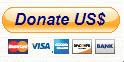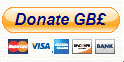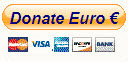## Extract data from Outlook e-mail message to Excel

It is a frequent requirement to extract data from similar e-mail messages. Some time ago I prepared a page on extracting to Word. This page covers the extraction (and automatic extraction) of e-mail data to an Excel workbook.

The process works by evaluating the 'lines' of a message for identifiable content, and then recovering the content from those 'lines' as required.

Messages may be simple text as shown below:or they may contain tables (or a combination of both). The following example shows the same data as the previous example, but in tabular form.The process needs to be tailored to the type of messages, being received. It is unlikely that the messages will be a mixture of both types.

The basic process will also work for messages that contain tables, as each cell of the table may be treated as a 'line'. The main difference is that table cells tend to contain only the required data and as this data is variable, it cannot be searched for, so it is necessary to evaluate the position of the required text with respect to 'lines' that contain related identifiable text.

To this end I began with an Outlook macro that will read the message and identify what is contained on each 'line'.

The following macro will read each line of the message in turn and display the content of the line, plus the contents of the two following lines (if any) complete with their line numbers in a message box. Note the line numbers which contain the required data, and if necessary the line number that precedes it that contains identifiable fixed data.

In the picture on the left, the data shown is a simple text format with fixed subject data and variable values on the same lines.

The picture on the right shows how this is read when the message is in tabular form. You will see that here the Customer Name subject is shown as 'Line 4' but the data relating to that subject is two lines later at 'Line 6', so by searching for the subject and adding two lines it is a simple matter to read the value required.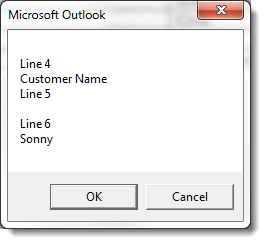Sub TestLines()
Dim olItem As Outlook.MailItem
Dim vText() As String
Dim sText As String
Dim i As Long
For Each olItem In Application.ActiveExplorer.Selection
sText = Replace(olItem.Body, Chr(160), Chr(32))
vText = Split(sText, Chr(13))
For i = 0 To UBound(vText)
sText = "Line " & i & vbCr & vText(i)
If i < UBound(vText) - 1 Then
sText = sText & vbCr & _
"Line " & i + 1 & vbCr & vText(i + 1)
End If
If i < UBound(vText) - 2 Then
sText = sText & vbCr & _
"Line " & i + 2 & vbCr & vText(i + 2)
End If
If MsgBox(sText, vbOKCancel) = vbCancel Then Exit Sub
Next i
Next olItem
End Sub

#### The main extraction macro

Having established how the data is located (and if providing questionnaires you can use the foregoing information to assist in their preparation, to simplify the data recovery process) we now need a macro to extract the data from the message itself.

Sub CopyToExcel(olItem As MailItem)
Dim xlApp As Object
Dim xlWB As Object
Dim xlSheet As Object
Dim vText As Variant
Dim sText As String
Dim vItem As Variant
Dim i As Long, j As Long
Dim rCount As Long
Dim bXStarted As Boolean
Const strWorkSheetName As String = "Sheet1"
Const strWorkBookName As String = "C:\Path\WorkBookName.xlsx" 'the path of the workbook
'Use FileExists function to determine the availability of the workbook
If Not FileExists(strWorkBookName) Then Exit Sub
'Get Excel if it is running, or open it if not
On Error Resume Next
Set xlApp = GetObject(, "Excel.Application")
If Err <> 0 Then
Set xlApp = CreateObject("Excel.Application")
bXStarted = True
End If
On Error GoTo 0

'Open the workbook to input the data
Set xlWB = xlApp.Workbooks.Open(strWorkBookName)
Set xlSheet = xlWB.Sheets("Sheet1")

'Process the message
With olItem
sText = olItem.Body
vText = Split(sText, Chr(13))
'Find the next empty line of the worksheet
rCount = xlSheet.Range("B" & xlSheet.Rows.Count).End(-4162).Row + 1

'Check each line of text in the message body
For i = UBound(vText) To 0 Step -1
If InStr(1, vText(i), "Source:") > 0 Then
vItem = Split(vText(i), Chr(58))
xlSheet.Range("A" & rCount) = Trim(vItem(1))
End If

If InStr(1, vText(i), "Customer Name:") > 0 Then
vItem = Split(vText(i), Chr(58))
xlSheet.Range("B" & rCount) = Trim(vItem(1))
End If

If InStr(1, vText(i), "Customer Email:") > 0 Then
vItem = Split(vText(i), Chr(58))
xlSheet.Range("C" & rCount) = Trim(vItem(1))
End If

If InStr(1, vText(i), "Customer Phone:") > 0 Then
vItem = Split(vText(i), Chr(58))
xlSheet.Range("D" & rCount) = Trim(vItem(1))
End If

If InStr(1, vText(i), "Move Date:") > 0 Then
vItem = Split(vText(i), Chr(58))
xlSheet.Range("E" & rCount) = Trim(vItem(1))
End If

If InStr(1, vText(i), "Origin City:") > 0 Then
vItem = Split(vText(i), Chr(58))
xlSheet.Range("F" & rCount) = Trim(vItem(1))
End If

If InStr(1, vText(i), "Origin State:") > 0 Then
vItem = Split(vText(i), Chr(58))
xlSheet.Range("G" & rCount) = Trim(vItem(1))
End If

If InStr(1, vText(i), "Origin Zip:") > 0 Then
vItem = Split(vText(i), Chr(58))
xlSheet.Range("H" & rCount) = Trim(vItem(1))
End If

If InStr(1, vText(i), "Destination City:") > 0 Then
vItem = Split(vText(i), Chr(58))
xlSheet.Range("I" & rCount) = Trim(vItem(1))
End If

If InStr(1, vText(i), "Destination State:") > 0 Then
vItem = Split(vText(i), Chr(58))
xlSheet.Range("J" & rCount) = Trim(vItem(1))
End If

If InStr(1, vText(i), "Destination Zip:") > 0 Then
vItem = Split(vText(i), Chr(58))
xlSheet.Range("K" & rCount) = Trim(vItem(1))
End If

If InStr(1, vText(i), "Vehicle Type:") > 0 Then
vItem = Split(vText(i), Chr(58))
xlSheet.Range("L" & rCount) = Trim(vItem(1))
End If

If InStr(1, vText(i), "Vehicle Year:") > 0 Then
vItem = Split(vText(i), Chr(58))
xlSheet.Range("M" & rCount) = Trim(vItem(1))
End If

If InStr(1, vText(i), "Vehicle Make:") > 0 Then
vItem = Split(vText(i), Chr(58))
xlSheet.Range("N" & rCount) = Trim(vItem(1))
End If

If InStr(1, vText(i), "Vehicle Model:") > 0 Then
vItem = Split(vText(i), Chr(58))
xlSheet.Range("O" & rCount) = Trim(vItem(1))
End If

If InStr(1, vText(i), "Vehicle Condition:") > 0 Then
vItem = Split(vText(i), Chr(58))
xlSheet.Range("P" & rCount) = Trim(vItem(1))
End If

If InStr(1, vText(i), "Comments:") > 0 Then
vItem = Split(vText(i), Chr(58))
xlSheet.Range("Q" & rCount) = Trim(vItem(1))
End If
Next i
xlWB.Save
End With
xlWB.Close SaveChanges:=True
If bXStarted Then
xlApp.Quit
End If
Set xlApp = Nothing
Set xlWB = Nothing
Set xlSheet = Nothing
End Sub

The macro above defines the workbook and worksheet as constants at the beginning. It also makes reference to a function (below) to determine if the workbook exists.

#### WWith this version of the macro, the worksheet must be pre-configured to collect the data and the details applied to the Constant settings.

Public Function FileExists(ByVal Filename As String) As Boolean
Dim nAttr As Long
On Error GoTo NoFile
nAttr = GetAttr(Filename)
If (nAttr And vbDirectory) <> vbDirectory Then
FileExists = True
End If
NoFile:
End Function

The main macro would normally be called as a 'script' from an Outlook rule that identifies the incoming messages to be processed. The process thereafter is completely automatic.#### If Outlook does not display the option to run a script in the above dialog, it is an indication that a security setting is in force. You can address this with a registry hack: 1. Click Start - Search, type ‘regedit’ (Note: there are no quotation marks in the command.) and press Enter. 2. Navigate to HKEY_CURRENT_USER\Software\Microsoft\Office\16.0\Outlook\Security. (where 16 is the Office version - here 2016) 3. Right-click a blank area, create a new DWORD Value named as ‘EnableUnsafeClientMailRules’ and set it to 1. Alternatively you could run the following macro to apply the registry setting described.

Sub SetOutlookSecurityKey()
Dim WSHShell As Object
Dim rKeyWord As String
Dim wVer As String
Dim RegKey As String
Dim strItem As String
strItem = "EnableUnsafeClientMailRules"
RegKey = "HKEY_CURRENT_USER\Software\Microsoft\Office\" & Application.Version & "\Outlook\Security\"
Debug.Print RegKey
End
Set WSHShell = CreateObject("WScript.Shell")
Start:
On Error Resume Next
Select Case rKeyWord
Case Is = "1"
MsgBox "Outlook Rules Security Check is Off"
Case Else
WSHShell.RegWrite RegKey & strItem, 1, "REG_DWORD"
MsgBox "Outlook Rules Security Check is Off"
End Select
lbl_Exit:
Exit Sub
End Sub

The script associated with the rule could also be run manually on a selected message (or messages), by calling it from a macro as follows:

Sub ExtractData()
Dim oItem As MailItem
If Application.ActiveExplorer.Selection.Count = 0 Then
MsgBox "No Items selected!", vbCritical, "Error"
Exit Sub
End If
For Each oItem In ActiveExplorer.Selection
CopyToExcel oItem
Next oItem
Set oItem = Nothing
End Sub

#### Excel

The basic code extracts as follows. Immediately you can see there are issues. In particular Column D is curtailed and includes the Text 'Hyperlink' which is not what is required.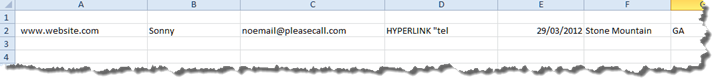The reason for this is that there are two colons in the required data and colon is used to split the subject from the data:

If InStr(1, vText(i), "Customer Phone:") > 0 Then
vItem = Split(vText(i), Chr(58))
xlSheet.Range("D" & rCount) = Trim(vItem(1))
End If

The line contains

Customer Phone: 8885551234

but the phone number part is formatted as a hyperlink and translates as shown in the result of the test macro:The line thus needs further processing before it can be applied to the worksheet.

If InStr(1, vText(i), "Customer Phone:") > 0 Then
'Split at the colon
vItem = Split(vText(i), Chr(58))
'Remove the subject and rebuild the string
For j = 1 To UBound(vItem)
If j < UBound(vItem) Then
End If
Next j
'Resplit at the quote marks
'Add the final part of the number to the worksheet
xlSheet.Range("D" & rCount) = Trim(vItem(UBound(vItem)))
End If

The result is then as shown in the following illustration:While on the subject of hyperlinks, it is likely that e-mail addresses will be hyperlinked in the messages and you would want to remove the hyperlinked text and extract only the e-mail address.

In the original macro the e-mail address is extracted with:

If InStr(1, vText(i), "Customer Email:") > 0 Then
vItem = Split(vText(i), Chr(58))
xlSheet.Range("C" & rCount) = Trim(vItem(1))
End If

If InStr(1, vText(i), "Customer Email:") > 0 Then
vItem = Split(vText(i), Chr(58))
For j = 1 To UBound(vItem)
Next j
End If
End If

#### Extract from table

Using the second message that contains the table as an example, this version does not have the colons so the lines do not require splitting. Thus using the following segment:

If InStr(1, vText(i), "Customer Name:") > 0 Then
vItem = Split(vText(i), Chr(58))
xlSheet.Range("B" & rCount) = Trim(vItem(1))
End If

we need to modify the code to take account of what we haveand here we see that the required value is two lines after the subject line, so the code needs to be modified as follows:

If InStr(1, vText(i), "Customer Name") > 0 Then
sAddr = Replace(vText(i + 2), Chr(10), "")
End If

Obviously the other segments will have to be modified to ensure that the correct data is applied to the appropriate column.

If you want to include data from the message itself, as opposed to from the body e.g. the date and time the message was received, you can add the following into the loop. Here the received time and date are added to columns R and S.

xlSheet.Range("R" & rCount) = Format(olItem.ReceivedTime, "dd/MM/yyyy") 'Date
xlSheet.Range("S" & rCount) = Format(olItem.ReceivedTime, "hh:mm") 'Time

If, having read through the foregoing, you feel that this is altogether too complicated, you will be heartened to learn that you can find a Word add-in that will extract the data from similar e-mail messages in Outlook to an Excel worksheet, to an Access table or to a Word document on this site. Click the link to view the page describing that add-in.

### Outlook to Excel

Some time ago, I posted a reply to a message in the MSDN programmers' forum demonstrating how to use a macro to extract data from similar e-mail messages to an Excel worksheet, using Outlook VBA.

This thread has proved popular, but through constant additions has become somewhat tortuous, so I have created this page to cover the salient points in a more accessible format.